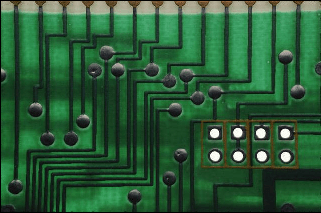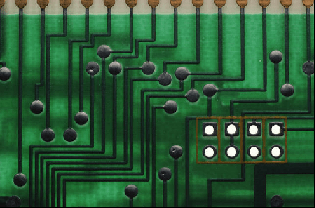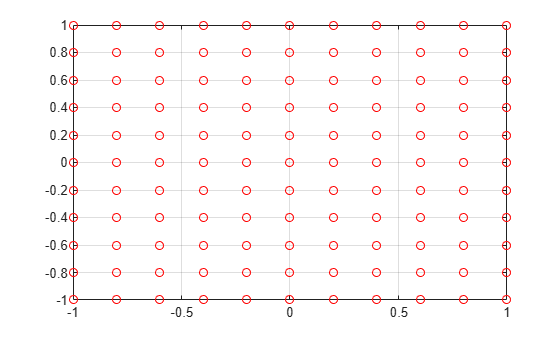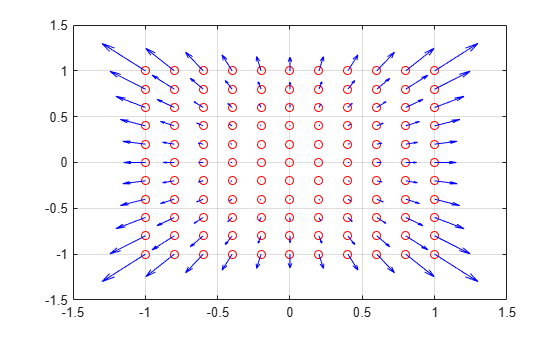# Developing an Algorithm for Undistorting an Image

This example develops a mathematical model using the Symbolic Math Toolbox to undistort an image and features a local function in the live script.

### Background

Any real world point $\mathit{P}\left(\mathit{X},\mathit{Y},\mathit{Z}\right)$can be defined with respect to some 3-D world origin.

Relative to a camera lens, this 3-D point can be defined as ${\mathit{p}}_{0}$, which is obtained by rotating and translating $\mathit{P}$.

${p}_{0}=\left({x}_{0},{y}_{0},{z}_{0}\right)$ = $R\phantom{\rule{0.16666666666666666em}{0ex}}P+t$

The 3-D point ${\mathit{p}}_{0}$ is then projected into the camera's image plane as a 2D point, (${\mathit{x}}_{1}$,${\mathit{y}}_{1}$).

${x}_{1}=\frac{{x}_{0}}{{z}_{0}}$ , ${y}_{1}=\frac{{y}_{0}}{{z}_{0}}$

When a camera captures an image, it does not precisely capture the real points, but rather a slightly distorted version of the real points which can be denoted (${\mathit{x}}_{2}$,${\mathit{y}}_{2}$). The distorted points can be described using the following function:

`${x}_{2}={x}_{1}\phantom{\rule{0.16666666666666666em}{0ex}}\left(1+{k}_{1}\phantom{\rule{0.16666666666666666em}{0ex}}{r}^{2}+{k}_{2}\phantom{\rule{0.16666666666666666em}{0ex}}{r}^{4}\right)+2\phantom{\rule{0.16666666666666666em}{0ex}}{p}_{1}\phantom{\rule{0.16666666666666666em}{0ex}}{x}_{1}\phantom{\rule{0.16666666666666666em}{0ex}}{y}_{1}+{p}_{2}\phantom{\rule{0.16666666666666666em}{0ex}}\left({r}^{2}+2\phantom{\rule{0.16666666666666666em}{0ex}}{{x}_{1}}^{2}\right)$`

`${y}_{2}={y}_{1}\phantom{\rule{0.16666666666666666em}{0ex}}\left(1+{k}_{1}\phantom{\rule{0.16666666666666666em}{0ex}}{r}^{2}+{k}_{2}\phantom{\rule{0.16666666666666666em}{0ex}}{r}^{4}\right)+2\phantom{\rule{0.16666666666666666em}{0ex}}{p}_{2}\phantom{\rule{0.16666666666666666em}{0ex}}{x}_{1}\phantom{\rule{0.16666666666666666em}{0ex}}{y}_{1}+{p}_{1}\phantom{\rule{0.16666666666666666em}{0ex}}\left({r}^{2}+2\phantom{\rule{0.16666666666666666em}{0ex}}{{y}_{1}}^{2}\right)$`

where:

${\mathit{k}}_{1}$, ${\mathit{k}}_{2}$ = radial distortion coefficients of the lens

${\mathit{p}}_{1}$, ${\mathit{p}}_{2}$ = tangential distortion coefficients of the lens

`$r=\sqrt{{{x}_{1}}^{2}+{{y}_{1}}^{2}}$`

### Distortion

An example of lens distortion is shown below; original distorted image (left) and undistorted image (right).Note the curvature of the lines toward the edges of the first image. For applications such as image reconstruction and tracking, it is important to know the real world location of points. When we have a distorted image, we know the distorted pixel locations (${\mathit{x}}_{2}$,${\mathit{y}}_{2}$). It's our goal to determine the undistorted pixel locations (${\mathit{x}}_{1}$,${\mathit{y}}_{1}$) given (${\mathit{x}}_{2}$,${\mathit{y}}_{2}$) and the distortion coefficients of the particular lens.

While otherwise straightforward, the nonlinear nature of the lens distortion makes the problem challenging.

### Define distortion model

We begin by defining our distortion model:

```% Parameters syms k_1 k_2 p_1 p_2 real syms r x y distortionX = subs(x * (1 + k_1 * r^2 + k_2 * r^4) + 2 * p_1 * x * y + p_2 * (r^2 + 2 * x^2), r, sqrt(x^2 + y^2))```
`distortionX = ${p}_{2} \left(3 {x}^{2}+{y}^{2}\right)+x \left({k}_{2} {\left({x}^{2}+{y}^{2}\right)}^{2}+{k}_{1} \left({x}^{2}+{y}^{2}\right)+1\right)+2 {p}_{1} x y$`
`distortionY = subs(y * (1 + k_1 * r^2 + k_2 * r^4) + 2 * p_2 * x * y + p_1 * (r^2 + 2 * y^2), r, sqrt(x^2 + y^2))`
`distortionY = ${p}_{1} \left({x}^{2}+3 {y}^{2}\right)+y \left({k}_{2} {\left({x}^{2}+{y}^{2}\right)}^{2}+{k}_{1} \left({x}^{2}+{y}^{2}\right)+1\right)+2 {p}_{2} x y$`

Radial Distortion ${\mathit{k}}_{1}=0$

We plot a grid of pixel locations assuming our lens has a radial distortion coefficient ${\mathit{k}}_{1}=0$. Note that distortion is smallest near the center of the image and largest near the edges.

```% Set Parameters parameters = [k_1 k_2 p_1 p_2]; parameterValues = [0 0 0 0]; plotLensDistortion(distortionX,distortionY,parameters,parameterValues)```
```spacing = 0.2000 ```
`distortionX = $x$`
`distortionY = $y$`Radial Distortion ${\mathit{k}}_{1}=0.15$

Explore the sensitivity to changes in ${\mathit{k}}_{1}$.

```% Set Parameters parameters = [k_1 k_2 p_1 p_2]; parameterValues = [0.15 0 0 0]; plotLensDistortion(distortionX,distortionY,parameters,parameterValues)```
```spacing = 0.2000 ```
```distortionX =  $x \left(\frac{3 {x}^{2}}{20}+\frac{3 {y}^{2}}{20}+1\right)$```
```distortionY =  $y \left(\frac{3 {x}^{2}}{20}+\frac{3 {y}^{2}}{20}+1\right)$```### Calculate Inverse Distortion Model

Given a camera's lens distortion coefficients and a set of distorted pixel locations (${\mathit{x}}_{2}$,${\mathit{y}}_{2}$), we want to be able to calculate the undistorted pixel locations (${\mathit{x}}_{1}$,${\mathit{y}}_{1}$). We will look at the specific case where all distortion coefficients are zero except for ${\mathit{k}}_{1}$ which equals 0.2.

We begin by defining the distortion coefficients

```syms X Y positive eq1 = X == distortionX```
`eq1 = $X={p}_{2} \left(3 {x}^{2}+{y}^{2}\right)+x \left({k}_{2} {\left({x}^{2}+{y}^{2}\right)}^{2}+{k}_{1} \left({x}^{2}+{y}^{2}\right)+1\right)+2 {p}_{1} x y$`
`eq2 = Y == distortionY`
`eq2 = $Y={p}_{1} \left({x}^{2}+3 {y}^{2}\right)+y \left({k}_{2} {\left({x}^{2}+{y}^{2}\right)}^{2}+{k}_{1} \left({x}^{2}+{y}^{2}\right)+1\right)+2 {p}_{2} x y$`

We define the distortion equations for given distortion coefficients, and solve for the undistorted pixel locations (${\mathit{x}}_{1}$,${\mathit{y}}_{1}$).

```parameters = [k_1 k_2 p_1 p_2]; parameterValues = [0.2 0 0 0]; eq1 = expand(subs(eq1, parameters, parameterValues))```
```eq1 =  $X=\frac{{x}^{3}}{5}+\frac{x {y}^{2}}{5}+x$```
`eq2 = expand(subs(eq2, parameters, parameterValues))`
```eq2 =  $Y=\frac{{x}^{2} y}{5}+\frac{{y}^{3}}{5}+y$```
`Result = solve([eq1, eq2], [x,y], 'MaxDegree', 3,'Real',true)`
```Result = struct with fields: x: (X*(((5*Y^3)/(2*(X^2 + Y^2)) + ((25*Y^6)/(4*(X^2 + Y^2)^2) + (125*... y: ((5*Y^3)/(2*(X^2 + Y^2)) + ((25*Y^6)/(4*(X^2 + Y^2)^2) + (125*Y^6)... ```

Since element 1 is the only real solution, we will extract that expression into its own variable.

`[Result.x Result.y]`
```ans =  ```

Now we have analytical expressions for the pixel locations X and Y which we can use to undistort our images.

### Function for drawing the lens distortion

```function plotLensDistortion(distortionX,distortionY,parameters,parameterValues) % distortionX is the expression describing the distorted x coordinate % distortionY is the expression describing the distorted y coordinate % k1 and k2 are the radial distortion coefficients % p1 and p2 are the tangential distortion coefficients syms x y % This is the grid spacing over the image spacing = 0.2 % Inspect and parametrically substitute in the values for k_1 k_2 p_1 p_2 distortionX = subs(distortionX,parameters,parameterValues) distortionY = subs(distortionY,parameters,parameterValues) % Loop over the grid for x_i = -1:spacing:1 for y_j = -1:spacing:1 % Compute the distorted location xout = subs(distortionX, {x,y}, {x_i,y_j}); yout = subs(distortionY, {x,y}, {x_i,y_j}); % Plot the original point plot(x_i,y_j, 'o', 'Color', [1.0, 0.0, 0.0]) hold on % Draw the distortion direction with Quiver p1 = [x_i,y_j]; % First Point p2 = [xout,yout]; % Second Point dp = p2-p1; % Difference quiver(p1(1),p1(2),dp(1),dp(2),'AutoScale','off','MaxHeadSize',1,'Color',[0 0 1]) end end hold off grid on end```

## Support

#### Mathematical Modeling with Symbolic Math Toolbox

Get examples and videos